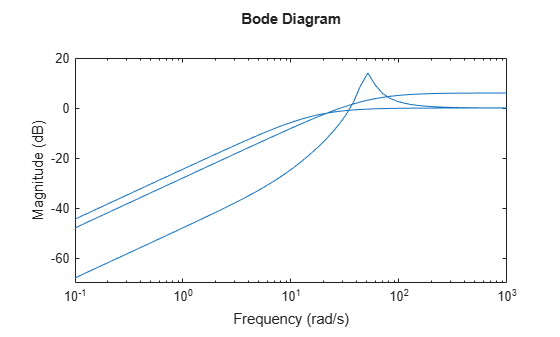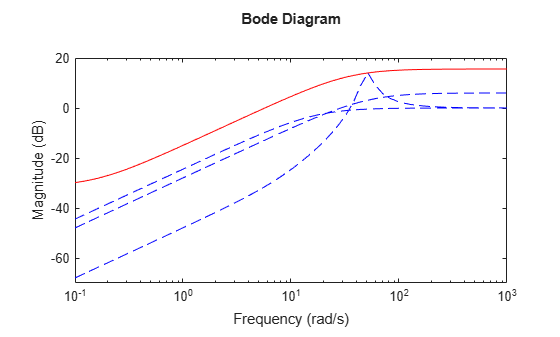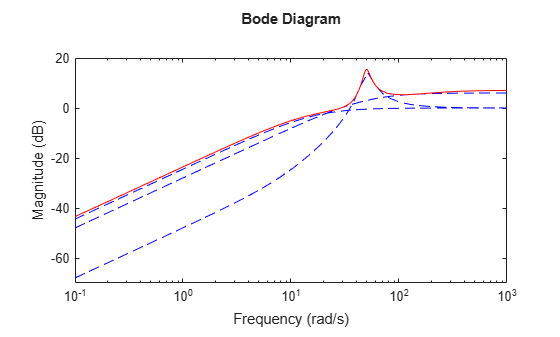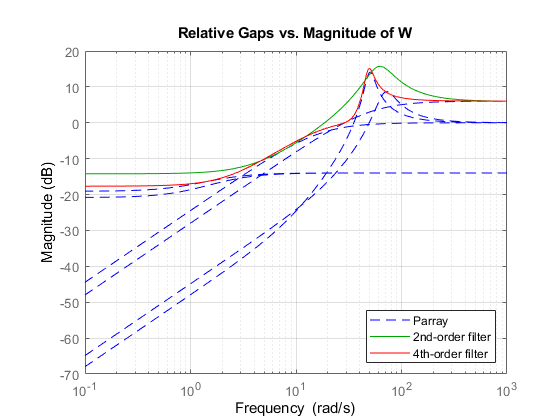# ucover

Fit an uncertain model to set of LTI responses

## Syntax

``usys = ucover(Parray,Pnom,ord)``
``usys = ucover(Parray,Pnom,ord1,ord2,utype)``
``[usys,info] = ucover(Parray,___)``
``[usys,info] = ucover(Pnom,info_in,ord1,ord2)``

## Description

example

````usys = ucover(Parray,Pnom,ord)` returns an uncertain model `usys` with nominal value `Pnom` and whose range of behaviors includes all responses in the LTI array `Parray`. The uncertain model structure is of the form $usys=Pnom\left(I+W\left(s\right)\Delta \left(s\right)\right)$, whereΔ is a `ultidyn` object that represents uncertain dynamics with unit peak gain.W is a stable, minimum-phase shaping filter of order `ord` that adjusts the amount of uncertainty at each frequency. For MIMO `Pnom`, W is diagonal, with orders of the diagonal elements given by `ord`.```
````usys = ucover(Parray,Pnom,ord1,ord2,utype)` returns an uncertain model with the structure specified by `utype`.`utype` = `'InputMult'` — Input multiplicative form, in which `usys = Pnom*(I + W1*Delta*W2)``utype` = `'OutputMult'` — Output multiplicative form, in which `usys = (I + W1*Delta*W2)*Pnom``utype` = `'Additive'` — Additive form, in which `usys = Pnom + W1*Delta*W2``Delta` represents uncertain dynamics with unit peak gain, and `W1` and `W2` are diagonal, stable, minimum-phase shaping filters with orders specified by `ord``1` and `ord``2`, respectively.```
````[usys,info] = ucover(Parray,___)` returns a structure `info` that contains information about the fit. You can use this syntax with any of the previous input-argument combinations.```
````[usys,info] = ucover(Pnom,info_in,ord1,ord2)` improves the fit using initial filter values in the `info` result. Supply new orders `ord`1 and `ord`2 for `W1` and `W2`. When you are trying different filter orders to improve the result, this syntax speeds up iteration by letting you reuse previously computed information.```

## Examples

collapse all

Fit an uncertain model to an array of LTI responses. The responses might be, for example, the results of multiple runs of acquisition of frequency response data from a physical system.

For the purpose of this example, generate the frequency response data by creating an array of LTI models and sampling the frequency response of those models.

```Pnom = tf(2,[1 -2]); p1 = Pnom*tf(1,[.06 1]); p2 = Pnom*tf([-.02 1],[.02 1]); p3 = Pnom*tf(50^2,[1 2*.1*50 50^2]); array = stack(1,p1,p2,p3); Parray = frd(array,logspace(-1,3,60));```

The frequency response data in `Parray` represents three separate data acquisition experiments on the system.

Plot relative errors between the nominal plant response and the three models in the LTI array.

```relerr = (Pnom-Parray)/Pnom; bodemag(relerr)```If you use a multiplicative uncertainty model structure, the magnitude of the shaping filter should fit the maximum relative errors at each frequency.

Try a 1st-order shaping filter to fit the maximum relative errors.

`[P,Info] = ucover(Parray,Pnom,1);`

`P` is an uncertain state-space (`uss`) model that captures the uncertainty as a `ultidyn` uncertain dynamics block.

`P.Uncertainty`
```ans = struct with fields: Parray_InputMultDelta: [1x1 ultidyn] ```

The `Info` structure contains other information about the fit, including the shaping filter. Plot the response to see how well the shaping filter fits the relative errors.

`bodemag(relerr,'b--',Info.W1,'r',{0.1,1000});`The plot shows that the filter `W1` is too conservative and exceeds the maximum relative error at most frequencies. To obtain a tighter fit, rerun the function using a 4th-order filter.

`[P,Info] = ucover(Parray,Pnom,4);`

Evaluate the fit by plotting the Bode magnitude plot.

`bodemag(relerr,'b--',Info.W1,'r',{0.1,1000});`This plot shows that for the 4th-order filter, the magnitude of `W1` closely matches the minimum uncertainty amount.

## Input Arguments

collapse all

Array of models to cover with a dynamically uncertain model, specified as an array of LTI models such as `tf`, `ss`, `zpk`, or `frd` models.

Nominal model of the uncertain model, specified as an LTI model such as a `tf`, `ss`, `zpk`, or `frd` model.

Filter order, specified as an integer, vector, or `[]`. The values in `ord` or in `ord`1 and `ord`2 specify the number of states of each diagonal entry of the shaping filters `W`, `W1`, and `W2`, respectively.

For the syntax `usys = ucover(Parray,Pnom,ord)`, specify `ord` as:

• A single integer, for SISO `Pnom`, or to use a scalar filter `W` for MIMO `Pnom`.

• A vector of length equal to the number of outputs in `Pnom`, to specify different orders for each diagonal entry of `W`.

• `[]`, to set `W = 1`.

For the syntax `usys = ucover(Parray,Pnom,ord1,ord2,utype)`, specify `ord1` and `ord2` as:

• A single integer, to us scalar filters for `W1` and `W2`.

• A vector, to specify different orders for each diagonal entry of `W1` and `W2`. The lengths of these vectors depend on the uncertainty model you specify in `utype`. The following table gives the lengths, where `Pnom` has `Nu` inputs and `Ny` outputs.

`utype``length(ord1)``length(ord2)`
`'InputMult'``Nu``Nu`
`'OutputMult'``Ny``Ny`
`'Additive'``Ny``Nu`
• `[]`, to set `W1 = 1` or ```W2 = 1```.

Uncertainty model, specified as one of the following.

• `'InputMult'` — Input multiplicative form, in which `usys = Pnom*(I + W1*Delta*W2)`

• `'OutputMult'` — Output multiplicative form, in which `usys = (I + W1*Delta*W2)*Pnom`

• `'Additive'` — Additive form, in which ```usys = Pnom + W1*Delta*W2```

`Delta` represents uncertain dynamics with unit peak gain, and `W1` and `W2` are diagonal, stable, minimum-phase shaping filters with orders specified by `ord``1` and `ord``2`, respectively.

Use additive uncertainty to model the absolute gaps between `Pnom` and `Parray`, and multiplicative uncertainty to model relative gaps.

For SISO models, input and output multiplicative uncertainty are equivalent. For MIMO systems with more outputs than inputs, the input multiplicative structure might be too restrictive and not adequately cover the range of models.

Details from previous `ucover` run, specified as a structure generated as the `info` output of the previous run. Use this input when calling `ucover` iteratively to improve fit results by trying different filter orders.

## Output Arguments

collapse all

Uncertain model, returned as a `uss` or `ufrd` model. The returned model is a `uss` model unless `Parray` or `Pnom` are frequency-response data (`frd`) models, in which case `usys` is a `ufrd` model.

`usys` has one uncertain element, a `ultidyn` block with the name given in the `DeltaName` field of the `info` output argument.

Information about the fit, returned as a structure containing the following fields.

FieldValue
`W1`

Fitted shaping filter `W` or `W1`, returned as a state-space (`ss`) model.

`W2`

Fitted shaping filter `W2`, returned as a state-space (`ss`) model.

`W1opt`

`W` or `W1` evaluated on a frequency grid, returned as an `frd` model.

`W2opt`

`W2` evaluated on a frequency grid, returned as an `frd` model.

`Ord1`

Orders of the diagonal elements of `W` or `W1`, returned as a scalar or vector. These values are the values you supply with the `ord` input argument.

`Ord2`

Orders of the diagonal elements of `W2`, returned as a scalar or vector. These values are the values you supply with the `ord` input argument.

`Type`

Uncertainty model used for the fit, returned as `'InputMult'`, `'OutputMult'`, or `'Additive'`.

`DeltaName`

Name of the `ultidyn` block of `usys` that represents the uncertainty model `Delta`, returned as a character vector.

`Residual`

Residuals of fit, returned as an array of `frd` models with the same array dimensions as `Parray`.

## Algorithms

`ucover` fits the responses of LTI models in `Parray` by modeling the gaps between `Parray` and the nominal response `Pnom` as uncertainty on the system dynamics. To model the frequency distribution of these unmodeled dynamics, `ucover` measures the gap between `Pnom` and `Parray` at each frequency on a grid, and selects shaping filters whose magnitude approximates the maximum gap.

To design the minimum-phase shaping filters `W1` and `W2`, the `ucover` command performs two steps:

1. Computes the optimal values of `W1` and `W2` on a frequency grid.

2. Fits `W1` and `W2` values with the dynamic filters of the specified orders using `fitmagfrd`.

The model structure $usys=Pnom\left(I+W\left(s\right)\Delta \left(s\right)\right)$ that you obtain using `usys = ucover(Parray,Pnom,ord)`, corresponds to `W1` = `W` and `W2` = 1.

For instance, the following figure shows the relative gap between the nominal response and six LTI responses, enveloped using a second-order shaping filter and a fourth-order filter.If you use the single-filter syntax `usys = ucover(Parray,Pnom,ord)`, the software sets the uncertainty to `W*Delta`, where `Delta` is a `ultidyn` object that represents unit-gain uncertain dynamics. This ensures that the amount of uncertainty at each frequency is specified by the magnitude of `W` and therefore closely tracks the gap between `Pnom` and `Parray`. In the above figure, the fourth-order filter tracks the maximum gap more closely and therefore yields a less conservative estimate of uncertainty.